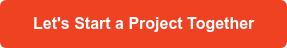# Going Back to Basics: Asymptotic Notation.

Tuesday 20 of November, 2018.
By Jonnathan Bartly

“It is better to invest in just 10 minutes of analyzing, than spending hours refactoring.”

How would you answer if I asked you: Is your code efficient? Are you using the right algorithm for problem solving?

If you spend a few minutes thinking about what you code, then you're on the right track. If you do not, it's time to go back to the basics and understand what Asymptotic Notation represents. But first let's review what an algorithm is.

An algorithm is a finite group of operations organized in a logical and orderly manner that allows you to solve a particular problem.

Now, let’s get to work!

#### Asymptotic Notation.

The Asymptotic Notation analyzes the time it takes an algorithm to execute. The execution time of an algorithm depends on how long it takes a computer to execute the lines of code of the algorithm. That depends on the speed of the computer, the programming language, and the compiler that translates the program, among other factors.

For example, let's say that an algorithm which runs with an input of size n, takes 6n2 + 100n + 300 machine instructions. The term 6n2 becomes larger than the rest of the terms 100n + 300 for n sufficiently large. Here is a graph that shows the values of 6n2 and 100n + 300 for values of n from 0 to 100:When we discard the least significant terms and the constant coefficients, we can focus on the really important part of an algorithm's execution time (the growth rate) without getting involved in details that complicate our understanding.

Asymptotic Notation is used when the constant coefficients and the least significant terms are discarded. We can divide the Asymptotic Notation into three forms: Big ThetaΘ notation, Big O notation, and Big OmegaΩ notation.

Let us begin!

#### Big ThetaΘ.Denote the size of the array (array.length) with n. The maximum number of times the for loop can be executed is n, The worst case occurs when the value being searched for is not present in the array. Every time we iterate, several things happen:

We compare guess with array.length. We also compare array[guess] with targetValue, and possibly return the value of guess and increase guess.

Each of these small calculations takes a constant amount of time every time it runs. If the for loop iterates n times, then the time for all iterations of n is c1⋅n, where c1 is the sum of the times for the calculations in one iteration of the loop. Now, we cannot say what the value of c1 is, because it depends on the speed of the computer, the programming language used, the compiler, and other factors.

But this is not all. We must include the initialization of guess to 0 to this code and possibly return -1 at the end. Let's call this time c2, which is also constant. Therefore, the total time for a linear search in the worst case is c1⋅n + c2.

As was already mentioned, the constant factors c1 and c2 tell us nothing about the growth rate of the execution time. The important part is that the execution time of a linear search in the worst case grows, as does the size of the array n. The notation we use for this execution time is Theta(n) or Θ(n). It reads "Big Theta of n" or simply "Theta of n".

When we say that a particular execution time is Theta(n), we are saying that once n is large enough, the execution time will be at least k1⋅n and at most k2 ⋅n, for some constants k1 and k2 . Keep the following graphic in mind when thinking about Theta(n):#### Big O.

We use the Big ThetaΘ notation to asymptotically limit the growth of a runtime within the range of constant factors above and below. However, there are times when we must limit only above.

In that case, when n is large enough, the execution time is, at most, k⋅f(n) for some constant k. Remember to think about this graph when dealing with a runtime that is O(f(n)):#### Big OmegaΩ.

Sometimes, we want to say that an algorithm takes, at least, a certain amount of time, without giving a higher bound. If a runtime is Omega(f(n)), then for a sufficiently large n, the runtime is at least k⋅f(n) for some constant k.#### Let's make an example!

Let's apply what we have just learned in real life, using an example of the Insertion sort algorithm we all know:

• The worst case: Θ(n2). - Disordered array.
• The best case: Θ(n). - Ordered array.
• The average case: Θ(n2) - Random array.

If you had to make a general statement that applies to all of the cases of the Insertion sort algorithm, you would have to say that it is executed in a time O(n2). You can’t say that it is executed in a time Θ(n2) in all cases, since the best case is executed in a time Θ(n). You can’t say that it is executed in a time Θ(n) in all cases either, since the execution time of the worst case is Θ(n2).

#### Conclusion.

Is your code efficient? Are you using the right algorithm for problem solving?

The Asymptotic Notation may seem a bit complicated to understand, but it is worth spending the necessary time to make the right decisions when implementing an algorithm and solving a problem. Give it a chance, who knows? (I know), the performance of your application may improve greatly.